# PSEB 10th Class Science Solutions Chapter 1 Chemical Reactions and Equations

Punjab State Board PSEB 10th Class Science Book Solutions Chapter 1 Chemical Reactions and Equations Textbook Exercise Questions and Answers.

## PSEB Solutions for Class 10 Science Chapter 1 Chemical Reactions and Equations

PSEB 10th Class Science Guide Chemical Reactions and Equations Textbook Questions and Answers

Question 1.
Which of the statements about the reaction below are incorrect?
2PbO(s) + C(s) → 2Pb(s) + CO2(g)
(b) Carbon dioxide is getting oxidised.
(c) Carbon is getting oxidised.
(d) Lead oxide is being reduced.
(i) (a) and (b)
(ii) (a) and (c)
(iii) (a), (b) and (c)
(iv) all.
(i) (a) and (b)

Question 2.
Fe2O3 + 2Al → Al2O3 + 2Fe
The above reaction is an example of a
(a) combination reaction.
(b) double displacement reaction.
(c) decomposition reaction.
(d) displacement reaction.
(d) displacement reaction.

Question 3.
What happens when dilute hydrochloric acid is added to iron filings?
(a) Hydrogen gas and iron chloride are produced.
(b) Chlorine gas and iron hydroxide are produced.
(c) No reaction takes place.
(d) Iron salt and water are produced.
(a) Hydrogen gas and iron chloride are produced.

Question 4.
What is a balanced chemical equation? Why should chemical equations be balanced?
Balanced chemical equation. It is a chemical equation in which number of atoms of each element are equal on both sides of the equation.

The chemical equation should be balanced because law of conservation of mass holds good i.e., the total mass of the reactants must be equal to the total mass of the products.Question 5.
Translate the following statements into chemical equations and then balance them :
(a) Hydrogen gas combines with nitrogen to form ammonia.
3H2 (g) + N2 → 2NH3 (g)

(b) Hydrogen sulphide gas burns in air to give water and sulphur dioxide.
2H2S (g) + 3O2 (g) → 2H2O (l) + 2SO2 (g)

(c) Barium chloride reacts with aluminium sulphate to give aluminium chloride and a precipitate of barium sulphate.
3BaCl2 + A12(SO4)3 → 2AlCl3 (aq) + 3BaSO4 (s)↓

(d) Potassium metal reacts with water to give potassium hydroxide and hydrogen gas.
2K (s) + 2H2O (l) → 2KOH (aq) + H2 (g)

Question 6.
Balance the following chemical equations :
(a) HNO3 + Ca(OH)2 → Ca(NO3)2 + H2O
2HNO3 + Ca(OH)2 → Ca(NO3)2 + 2H2O

(b) NaOH + H2SO4 → Na2SO4 + H2O
2NaOH + H2SO4 → Na2SO4 + 2H2O

(c) NaCl + AgNO3 → AgCl + NaNO3
NaCl + AgNO3 → AgCl + NaNO3

(d) BaCl2 + H2SO4 → BaSO4 + HCl
BaCl2 + H2SO4 → BaSO4 + 2HCl

Question 7.
Write the balanced chemical equations for the following reactions :
(a) Calcium hydroxide + Carbon dioxide → Calcium carbonate + Water
Ca(OH)2 + CO2 → CaCO3 + H2O

(b) Zinc + Silver nitrate → Zinc nitrate + Silver.
Zn + 2AgNO3 → Zn(NO3)2 + 2Ag

(c) Aluminium + Copper chloride → Aluminium chloride + Copper.
2Al + 3CuCl2 → 2AlCl3 + 3Cu

(d) Barium chloride + Potassium sulphate → Barium sulphate + Potassium chloride.
BaCl2 + K2SO4 → BaSO4 + 2KCl

Question 8.
Write the balanced chemical equation for the following reaction and identify the type of reaction in each case.
(a) Potassium bromide (aq) + Barium iodide (aq) → Potassium iodide (aq) + Barium bromide (s)
2KBr (aq) + Bal2 (aq) → 2KI (aq) + BaBr2 (aq) — Double displacement reaction

(b) Zinc carbonate (s) → Zinc oxide (s) + Carbon dioxide (g)
ZnCO3 (s) → ZnO (s) + CO2 (g) — Decomposition reaction

(c) Hydrogen (g) + Chlorine (g) → Hydrogen chloride (g)
H2(g) + Cl2 (g) → 2HCl(g) — Combination reaction

(d) Magnesium (s) + Hydrochloric acid (aq) → Magnesium chloride (aq) + Hydrogen (g)
Mg(s) + 2HCl(aq) → MgCl2 (aq) + H2 (g) — Displacement reaction

Question 9.
What is meant by exothermic and endothermic reactions? Give examples.
Or
Differentiate between exothermic and endothermic reactions.
Exothermic reaction. It is a chemical reaction in which heat energy is given out.
e.g. C (s) + O2 (g) → CO2 + Heat energy
NaOH (aq) + HCl (aq) → NaCl (aq) + H2O (l) + Heat energy

Endothermic reaction : It is a chemical reaction in which heat energy is absorbed,
e.g. N2 (g) + O2 (g) → 2NO (g) — Heat energy
C (S) + H2O (g) → CO + H2 (g) — Heat energy.

Question 10.
Why is respiration considered as an exothermic reaction? Explain.
During respiration oxidation of glucose occurs which produces heat energy.

Question 11.
Why are decomposition reactions called opposite of combination reactions? Write equations for these reactions.
During decomposition a single substance breaks down into two or more substances which is just the reverse of combination reaction.

Examples for decomposition reactions are :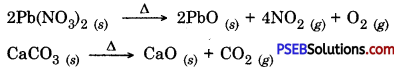Question 12.
Write one equation each for decomposition reactions where energy is supplied in the form of heat, light or electricity.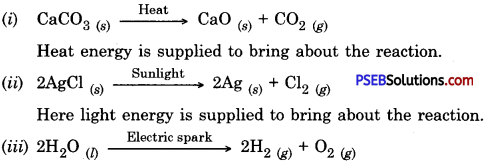Here electrical energy is supplied to bring about the reaction.

Question 13.
What is the difference between the displacement and double displacement reactions? Write equations for these reactions.
Displacement reaction: In this reaction a more active element displaces less active element from solution of its compound. Examples:
Fe (s) + CuSO4 (aq) → FeSO4 (aq) + Cu (s) ↓
Zn (s) + CuSO4 (aq) → ZnSO4 (aq) + Cu (s) ↓

Double displacement reaction: The reaction in which there is an exchange of ions between two reactants is called a double displacement reaction.
AgNO3 (aq) + NaCl (aq) AgCl (s) ↓ + NaNO3 (aq)
BaCl2 (aq) + Na2SO4 (aq) BaSO4 (s) ↓ + 2NaCl (aq)

Question 14.
In the refining of silver, the recovery of silver from silver nitrate solution involved displacement by copper metal. Write down the reaction involved.
Cu (s) + 2AgNO3 (aq) → Cu(NO3)2 (aq) + 2Ag ↓Question 15.
What do you mean by precipitation reaction? Explain by giving examples.
Precipitation reaction. A reaction in which an insoluble product or precipitate is produced is called precipitation reaction.
AgNO3 (aq) + NaCl (aq) → AgCl (s) ↓ + NaNO3 (aq)
BaCl2 (aq) + Na2SO4 (aq) → BaSO4 (s) ↓ + 2NaCl (aq)

Question 16.
Explain the following in terms of gain or loss of oxygen with two examples each :
(a) Oxidation (b) Reduction.
(a) Oxidation: A chemical reaction in which a substance gains oxygen or loses hydrogen is called oxidation.
Examples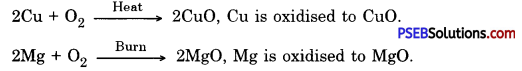(b) Reduction: A chemical reaction in which a substance loses oxygen or gains hydrogen is called reduction.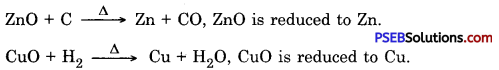Question 17.
A shiny brown coloured element ‘X’ on heating in air becomes black in colour. Name the element ‘X’ and the black coloured compound formed.
The element X is copper.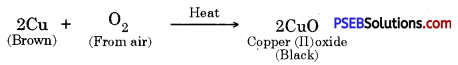Therefore the black coloured compound formed is copper (II) oxide (CuO).

Question 18.
Why do we apply paint on iron articles?
The iron articles can be protected from rusting by applying paint on them so that the iron surface does not come in contact with air (or oxygen) and moisture which causes rusting.

Question 19.
Oil and fat containing food items are flushed with nitrogen. Why?
This is because food items are prevented from oxidation by oxygen or air.

Question 20.
Explain the following terms with one example each :
(a) Corrosion
Corrosion: The slow eating up of metals by the action of air and moisture on their surfaces is called corrosion. For example, iron undergoes corrosion or rusting in the presence of moist air.

(b) Rancidity.
Rancidity: When fats and oils or food containing oils and fats get oxidised with air or oxygen, their smells and tastes change, This process is called rancidity. For example, the packet containing potato chips is flushed with nitrogen gas to avoid rancidity.

Science Guide for Class 10 PSEB Chemical Reactions and Equations InText Questions and Answers

Question 1.
Why should a magnesium ribbon be cleaned before burning in air?
Magnesium ribbon should be cleaned before burning to remove the protective layer of basic magnesium carbonate from its surface.

Question 2.
Write the balanced equation for the following chemical reactions.
(i) Hydrogen + Chlorine → Hydrogen chloride
H2 + Cl2 → 2HCl

(ii) Barium chloride + Aluminium Sulphate → Barium Sulphate + Aluminium Chloride
3BaCl2 + Al2(SO4)3 → 3BaSO4 + 2AlCl3

(iii) Sodium + Water → Sodium hydroxide + Hydrogen
2Na + 2H2O → 2NaOH + H2

Question 3.
Write a balanced chemical equation with state symbols for the following reactions.
(i) Solutions of barium chloride and sodium sulphate in water react to give insoluble sulphate and the solution of sodium chloride.
BaCl2(aq) + Na2SO4(aq) → BaSO4(s) + 2NaCl(aq)

(ii) Sodium hydroxide solution (in water) reacts with hydrochloric acid solution (in water) to produce sodium chloride and water.
NaOH (aq) + HCl (aq) → NaCl (aq) + H2O (l)Question 4.
A solution of substance “X’ is used for white washing.
(i) Name the substance X’and write its formula.
X is calcium oxide (quick lime) and its formula is CaO.

(ii) Write the reaction of the substance X named in (i) above with water.
CaO (s) + H2O(l) → Ca(OH)2 (aq)

Question 5.
Why is the amount of gas collected in one of the test tubes in activity 1.7 double of the amount collected in the other? Name this gas.
When electric current is passed through acidulated water, the reaction taking place is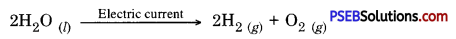Therefore hydrogen and oxygen produced are in the ratio 2 : 1 by volume. Hence volume of gas collected in one test tube is double the volume of gas collected in the other tube and this gas is hydrogen.

Question 6.
Why does the colour of copper sulphate solution change, when an iron nail is dipped in it?
This is because iron displaces copper from copper sulphate solution.
Therefore, the concentration of copper sulphate solution decreases and blue colour of solution gradually fades away.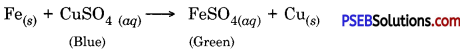The concentration of copper sulphate solution decreases as ferrous sulphate is produced.

Question 7.
Give an example of a double displacement reaction other than the one given in the activity 1.10 given in textbook